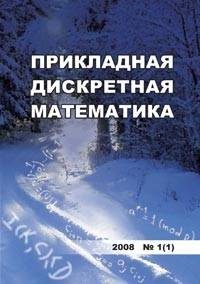# О разрешимости регулярных уравнений в многообразии метабелевых групп

We study the solvability of equations over groups within a given variety or another class of groups. The classes of nilpotent and solvable groups were considered as main classes to investigate from such point of view. The natural analogues of the famous Kervaire - Laudenbach and Levin conjectures were raised to the challenge. It was also noted that the "solvable" version of the known theorem by Brodskii is not true. In this paper, for each n Е N, n ^ 2, we prove that every regular equation over the free metabelian group Mn is solvable in the class M of all metabelian groups. Moreover, there is a metabelian group Mn that contains a solution of every unimodular equation over Mn. These results are extended to the class of rigid metabelian groups. Also, we give an example showing that there exists an equation over a locally indicable torsion-free metabelian group G that has no solution in any solvable overgroup of G. It follows that solvable versions of the Levin conjecture are not true. Another example presents an unimodular equation over a locally indicable torsion-free metabelian group G that has no solution in any metabelian overgroup of G. Hence, the Kervaire - Laudenbach conjecture is not valid for the variety of all metabelian groups. We prove that there is an unimodular equation over a finite metabelian group G that has no solutions in any finite metabelian overgroup of G. This means that analog of the famous theorem by Gerstenhaber and Rothaus (about solvability of each unimodular equation over a finite group G in some finite overgroup of G) is not valid for the class of finite metabelian groups.

## Ключевые слова

Kervaire-Laudenbach conjecture, Levin conjecture, solvable group, metabelian group, rigid group, nilpotent group, locally indicable group, regular equation, solvability over group, гипотеза Кервер-Лауденбаха, гипотеза Левина, разрешимая группа, метабелевая группа, нильпотентная группа, жесткая группа, локально отображаемая группа, регулярное уравнение, разрешимость по группе

## Авторы

 ФИО Организация Дополнительно E-mail Романьков Виталий Анатольевич Омский государственный университет им. Ф. М. Достоевского доктор физико-математических наук, профессор romankov48@mail.ru
Всего: 1

## Ссылки

Noskov G. A, Remeslennikov V. N., and Roman'kov V. A. Infinite groups. J. Sov. Math., 1982, vol. 18, pp. 669-735.
Remeslennikov V. N. and Roman'kov V. A. Model-theoretic and algorithmic questions in group theory. J. Sov. Math., 1985, vol.31, pp. 2887-2939.
Kharlampovich O. and Myasnikov A. Equations and Algorithmic Problems in Groups. Publicacoes Math., IMPA, Brazil, 2008. 79 p.
Roman'kov V. Equations over groups. Groups, Complexity, Cryptology, 2012, vol.4, pp.191-239.
Gerstenhaber V. and Rothaus O. S. The solutions of sets of equations in groups. Proc. Nat. Acad. Sci.U.S., 1962, vol.48, pp. 1951-1953.
Rothaus O. S. On the non-triviality of some group extensions given by generators and relators. Ann. Math., 1977, vol. 106, pp. 599-612.
Klyachko A. Funny property of sphere and equations over groups. Comm. Algebra, 1993, vol. 21, pp. 2555-2575.
Brodskii S. D. Equations over groups and groups with a single defining relation. Russian Math. Surveys, 1980, vol. 35, pp. 165.
Brodskii S. D. Equations over groups and groups with a single defining relator. Siberian Math. J., 1984, vol. 25, pp. 84-103.
Howie J. On locally indicable groups. Math. Z., 1982, vol. 180, pp. 445-461.
Gersten S. M. Reducible diagrams and equations over groups. In: Gersten, S.M., ed. Essays in Group Theory. MSRI Publ., Springer Verlag, 1985, vol.8, pp. 15-73.
Krstic S. Systems of equations over locally p-indicable groups. Invent. Math., 1985, vol.81, pp. 373-378.
Timoshenko E. I. Endomorfizmy i universal'nye teorii razreshimykh grupp [Endomorphisms and universal theories of solvable groups]. Novosibirsk, NSTU Publishers, 2011. 327p. (NSTU Monographs Ser.) (in Russian).
Baumslag G. Some theorems on the free groups of certain product varieties. J. Combinatorial Theory, 1967, vol.2, pp. 77-99.
Myasnikov A. and Romanovskiy N. S. Krull dimension of solvable groups. J. Algebra, 2010, vol.324, pp. 2814-2831.
Romanovskiy N. S. Divisible rigid groups. Algebra and Logic, 2008, vol. 47, pp. 426-434.